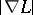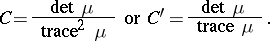# Corner detection

(diff) ← Older revision | Latest revision (diff) | Newer revision → (diff)

A processing stage in computer vision algorithms, aimed at detecting and classifying the nature of junctions in the image domain. A main reason why corner detection is important is that junctions provide important cues to local three-dimensional scene structure [a1].

The presumably most straightforward method for detecting corners is by intersecting nearby edges. While this approach may give reasonable results under simple conditions, it relies on edge detection as a pre-processing stage and suffers from inherent limitations. For example, not all corners arise from intersections of straight edges. In addition, edge detectors have problems at junctions.

One way of detecting junctions directly from image intensities consists of finding points at which the gradient magnitudeand the curvature of level curvesassume high values simultaneously [a2], [a3]. A special choice is to consider the product of the level curve curvature and the gradient magnitude raised to the power three. This is the smallest value of the exponent that leads to a polynomial expression for the differential invariantMoreover, spatial extrema of this operator are preserved under affine transformations in the image domain, which implies that corners with different opening angles are treated in a qualitatively similar way. Specifically, spatial maxima of the square of this operator are regarded as candidate corners [a4], [a5].

When implementing this corner detector in practice, the computation of the discrete derivative approximations are preceded by a Gaussian smoothing step (see Scale-space theory; Edge detection).

Another class of corner detectors [a6], [a7] is based on second-moment matrices [a5]:and corner features are defined from local maxima in a strength measure such asAlso, this feature detector responds to local curvature properties of the intensity landscape [a8].

How to Cite This Entry:
Corner detection. Encyclopedia of Mathematics. URL: http://encyclopediaofmath.org/index.php?title=Corner_detection&oldid=12237
This article was adapted from an original article by Tony Lindeberg (originator), which appeared in Encyclopedia of Mathematics - ISBN 1402006098. See original article